## research papersBIOLOGICALCRYSTALLOGRAPHY
ISSN: 1399-0047
Volume 58| Part 11| November 2002| Pages 1907-1914

## Towards the automated evaluation of crystallization trials

aYork Structural Biology Laboratory, Chemistry Department, University of York, Heslington, York YO10 5DD, England
*Correspondence e-mail: julie@ysbl.york.ac.uk

(Received 5 February 2002; accepted 12 September 2002)

A method to evaluate images from crystallization experiments is described. Image discontinuities are used to determine boundaries of artifacts in the images and these are then considered as individual objects. This allows the edge of the drop to be identified and any objects outside this ignored. Each object is evaluated in terms of a number of attributes related to its size and shape, the curvature of the boundary and the variance in intensity, as well as obvious crystal-like characteristics such as straight sections of the boundary and straight lines of constant intensity within the object. With each object in the image assigned to one of a number of different classes, an overall report can be given. The objects to be considered have no predefined shape or size and, although one may expect to see straight edges and angles in a crystal, this is not a prerequisite for diffraction. This means there is much overlap in the values of the variables expected for the different classes. However, each attribute gives some information about the object in question and, although no single attribute can be expected to correctly classify an image, it has been found that a combination of classifiers gives very good results.

### 1. Introduction

The number of putative protein sequences determined by worldwide DNA-sequencing efforts in recent years now vastly exceeds the rate at which protein structures can be analyzed experimentally. Although methods are being developed to predict protein structure from the sequence alone, accurate experimentally determined molecular structures are necessary for structure-based functional studies and effective drug design. Crystallography can reliably provide the answer to many such questions when suitable crystals are obtained. Improvements to beamline optics and the intense highly focused X-rays available at synchrotron sources now allow the use of flash-frozen micrometre-sized crystals. Along with advances in protein expression and purification, the automation of microcrystallization is an essential tool for high-throughput protein crystallography. Robotic systems capable of performing thousands of crystallization experiments a day have been developed and are already in use in a number of laboratories (Stevens, 2000). The results from each of these experiments must be recorded and assessed routinely and automatically. So far, the available detection software can only indicate the presence or absence of crystal-like objects and results must be verified by manual inspection (Rupp, 2000). The difficulty is intrinsic to the problem: the size and morphology of crystals can vary greatly and it is vital to develop software that can also identify other phenomena in the drop. Microcrystals and aggregates, thin plates, clusters of needles and crystalline precipitates all indicate conditions that could be optimized and must be recognized as well as large single crystals. Cracks and other irregularities in crystals also have to be dealt with.

### 2. Image analysis

The edges of a structure are often the most important features in pattern recognition, as demonstrated by our ability to recognize an object from a rough line drawing, and the nature of crystal growth makes edge detection an obvious choice for identifying the presence of crystals. Traditionally, edges are defined as pixel-intensity discontinuities within an image and most edge-detection methods identify edge points from the extrema of the first- or second-order derivatives of the image (see, for example, Marr & Hildreth, 1980).

An image can be considered as a two-dimensional function, f(xy), with the pixels classified on a greyscale from 0 to 255. Fig. 1shows how an image can be transformed to a three-dimensional plot, making the idea of gradient or slope intuitively obvious.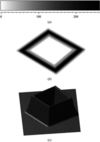Figure 1 (a) The numerical scale assigned to the greyscale of an image. A two-dimensional image is shown in (b), with the corresponding three-dimensional plot in (c).

The gradient is related to the slope of the surface at every point and is defined by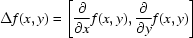where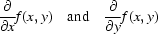denote the partial derivatives with respect to x and to y. The gradient is a vector quantity with a magnitude, indicating the steepness of the slope, given by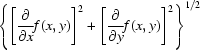and a direction defined by the angle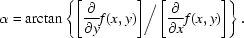This gives the direction of the greatest uphill slope and the amount of the slope in that direction. The plot of gradient magnitudes in Fig. 2(b) shows that sudden changes in intensity give rise to sharp edges' corresponding to the steepest slopes. As well as the important outlines of an object, the small details that may be considered as texture will also produce edges on a different scale. This is more obvious in Fig. 3, which shows Fig. 2(b) after sharpening. Some cutoff level for the gradient magnitude must be chosen before attempting to group the edges together into objects for classification. Different edge-detection algorithms have been tried and it was found that there was very little difference between the results. In the current work, a simple Sobel operator is used.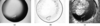Figure 2 The gradient magnitudes for the image in (a) are shown in (b). The darkest points here correspond to the steepest gradient. The direction of the gradient at each point is shown in (c), where the direction is plotted as an angle between 0 and 360°. The sharp change from black to white seen along the edge of the drop is merely owing to the change in angle between 0 and 360°.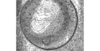Figure 3 The same gradient magnitudes as shown in Fig. 2(a), after sharpening, in order to show the smaller scale edges.

Effects such as light and shadow can blur some informative edges, causing them to fall below the cutoff level. However, as the direction of maximum gradient is always perpendicular to an edge, it can also be used to help define edges. For a straight-line edge, such as that along the crystal face in Fig. 2, the gradient direction is constant, whereas for the circular edge of the drop the direction changes gradually. Thus, the gradient direction can be used to accept points below the cutoff level when an edge is being followed.

The identification of the edge of the drop is particularly important when the crystallization drop is small in comparison to the overall image, as is often the case with images from robotic experiments. As any line perpendicular to a tangent of a circle passes through the centre of the circle, the crossing point of such lines identifies the centre of the circle (Fig. 4a). Although the edge of a crystallization drop is often far from a perfect circle, the same idea can be applied to roughly circular objects by considering lines perpendicular to the tangent at every point on the boundary (Fig. 4b). In fact, the direction of these lines is given by the direction of the gradient at each boundary point. An estimate for the position of the centre is obtained by averaging the positions of the points where these lines cross and from this an average radius can be calculated. The variation in the crossing points gives an indication of the circularity of the object. The method will also identify incomplete circles, provided that at least 50% of the drop edge is captured in the image. Once the edge of the drop is identified, attention can be focused inside the drop and any objects outside this can be ignored. The edges of the well, for example, should be eliminated before a cutoff level is applied.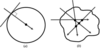Figure 4 (a) Any line perpendicular to the tangent of a circle passes through the centre of a circle. For the roughly circular object shown in (b), lines in the direction of the steepest gradient do not cross at a single point, but the crossing points can be used to estimate the centre and provide a measure of circularity.

The initial cutoff level determines the groups of pixels to be considered as objects. If this is too high objects will be missed and if it is too low objects can become very large and, for example, crystals can be lost amongst precipitate. Similarly, objects very close to the edge of the drop may be discarded along with the edge of the drop. This can be overcome by varying the cutoff level, although the time taken to evaluate each image is then increased.

Once the important edges in an image have been identified, they are grouped together into individual objects by connectivity. Fig. 5shows the pixels with gradient magnitude above the cutoff level in black; it can be seen that the connected sets of pixels form separate objects. The objects are then evaluated in terms of a number of different attributes or classifiers that are used to determine a class for each object. At present, each object is assigned to one of the following five classes.

 XTAL: single crystals. PILE: overlapping crystals or clusters. INT: promising conditions such as interesting precipitate or microcrystals. SKIN: objects arising from skin on the drop as well as various lighting effects. JUNK: other unfavourable outcomes.
The two classes PILE and SKIN have many variables with common values and having separate categories for these objects aids classification. Hence, piles of crystals are classed separately from single crystals and objects arising from skin on the drop are not included among other unfavourable outcomes.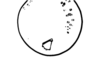Figure 5 The objects found from the image in Fig. 2(a).

### 3. The classification variables

In order to assign an object to one of the five classes, numerical values are associated with certain features. For example, the total area covered by an object and the length of its boundary can both be evaluated in terms of the number of pixels. The ratio of these two numbers gives a single value that gives some information about the shape of the object. No single characteristic can be expected to distinguish between all the different classes and a number of distinct classifiers are evaluated for each object. The classifiers used are described in the following sections and are summarized in Table 1.

 Table 1Summary of the classifiers currently used in the method and described in the text
Classifiers Description
X1X4 Lines of constant intensity.
X5 Ratio of minimum box area to object area.
X6 Ratio of object's area to perimeter.
X7 Rectangularity of minimal box.
X8X9 Variation in intensity with the object (in both the original image and in the edge image).
X10X11 Measures of straightness and curvature of the boundary (using chain codes).
X12X13 Shape descriptors from the boundary of the object.

#### 3.1. Straight lines

An obvious candidate for classification is the presence of straight lines and regular angles in crystals. It can be seen in Fig. 5that even for such a good crystal, much of the boundary is not straight. For this reason, the variation in the greyscale of the edge image is considered and lines of constant intensity anywhere within the object are sought. The length of the line is important and lines of different length related to the overall size of the object are used. Of course, it is possible to find straight lines of constant intensity across non-crystalline objects, although the angles between lines and indeed the angles of the lines themselves can give an indication as to the type of object. For example, in Fig. 6, the edge of the crystallization drop (not eliminated in this case for demonstration) has created an object in which many lines of constant intensity have been found. There are lines at virtually every possible angle (to the horizontal) within this object, whereas the crystal only has lines at limited angles. This cannot be used as a definitive test, however, as other objects, such as crystalline aggregates, give similar results. In fact, none of the classifiers would individually correctly identify objects, but a vector of values obtained from a combination of classifiers can be used successfully.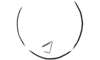Figure 6 The straight lines for which the intensity in Fig. 2(b) remains constant. Here, the edge of the drop has not been eliminated in order to demonstrate the fact that the algorithm also picks up such things. However, for this image it is easily identified.

#### 3.2. Shape

Skin on the drop and various lighting effects also give rise to objects with straight lines and angles characteristic of crystals. Generally, the shape of such objects immediately distinguishes them from crystals and can therefore be used for classification. Typically, a large area of the drop is covered by a relatively small number of pixels and boundaries tend to be very long in comparison to the area of the object. Consider the minimal rectangular box needed to completely enclose an object. The ratio of the number of pixels in this box to the number of pixels in the object will have a different value for different shapes (see Fig. 7a). Furthermore, we can say something about the shape of the minimal box, i.e. how rectangular it is. The quantity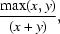where x and y are the lengths of the sides of the rectangle, will give a value of 0.5 when x = y, i.e. when the minimal box is square. However, if x is very small in comparison to y and we have a very long thin rectangle, then we obtain a value close to 1.0. Fig. 7(b) shows a shape typical of precipitate that has been grouped together as a single object. Here, there are a large number of pixels on the boundary in comparison to the overall size of the object, whereas the crystal-like object in the figure has a smaller perimeter-to-area ratio.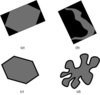Figure 7 The ratio of the minimal box area to the object area is different for different shapes: compare the back and grey areas in (a) for a crystal-like object and in (b) for an object typical of skin on the drop. The ratio of the area to perimeter of an object is also different for different shapes: compare the length of the black boundaries with the grey areas in (c) for the crystal-like object and in (d) for an object similar to those obtained for precipitate.

#### 3.3. Texture and contrast variation

Distinguishing good' precipitate from amorphous sludge is often a difficult problem even by eye (see Bergfors, 2000). However, any automated system which aims to evaluate the outcomes of crystallization experiments will not only need to recognize the presence or absence of crystals, but will also need to identify conditions that can be refined for crystal growth. Fig. 8shows that a light precipitate cannot be distinguished from microcrystals by shape. In both cases, we obtain objects with very long twisted boundaries as well as many very small objects. However, the original image shows very different variation in intensity across the pixels in the objects. Crystalline matter shows a much greater contrast than a dull precipitate. This is also true of the edge image, as the gradient magnitude varies more across crystalline objects. The variation in intensity, both in the original image and in the gradient magnitude, is used for classification.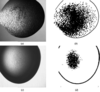Figure 8 Comparison of the objects in (b) from the image in (a) and the objects in (d) from the image in (c) shows that shape alone cannot distinguish between microcrystals and a dull precipitate.

#### 3.4. Curvature

In order to say something about the way in which the boundary of an object is changing, the pixels on the boundary need to be ordered. Chain codes (Freeman, 1961) provide a simple way of encoding the change in direction from one pixel to the next along the boundary. The direction from one pixel on the boundary to the next are associated with a number, ranging from 0 to 7, representing shifts of 0, 45, 90, 135, 180, 225, 270 and 315°, as shown in Fig. 9(a). The chain code for the boundary in Fig. 9(b), starting from the pixel indicated, is therefore given by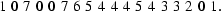Along the straight sections of the boundary, there is no change in the chain code. In fact, the differences between adjacent chain codes show how the boundary is changing. An estimate for the curvature at each point on the boundary is obtained by taking the chain code for this point and subtracting the chain code for the preceding point. Thus, the curvature for this boundary in is given by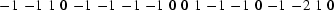[using 7 ≅ −1 (mod 8)]. The zeros correspond to the straight sections of the boundary, with the number of consecutive zeros indicating the length of that section. The parity is unimportant here, as it merely shows a convex or concave corner and it is the absolute value that matters. For example, the value of 2 towards the end of the boundary arises from the more acute corner here. The sum of these absolute values provides a value associated with the curvature and a measure of straightness is obtained by considering the consecutive zeros.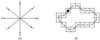Figure 9 In order to encode the boundary, a number is associated with each of the eight possible directions as shown in (a). In (b) an example of a boundary is shown for which the chain code is given in the text. The starting point for the chain code is indicated by a black spot.

#### 3.5. Boundary-shape descriptors

With the points on the boundary ordered, other classifiers that distinguish between shapes can be defined. Consider the graph of distances from some starting point on the boundary to every other point on that boundary in order. Figs. 10(a) and 10(b) show the graphs obtained for a circle and a rectangle. Whilst the graph for the rectangle is more angular, both shapes have graphs with only one maxima, whereas in Fig. 10(c) the graph for an object with a very twisted boundary has multiple extrema. Even small changes can lead to local extrema, but the difference between adjacent maxima and minima distinguishes large twists and turns from small changes on the boundary. This can be used to assign a numerical value to the object based on the shape of the boundary. Furthermore, the area under the graph obtained by integration can also be used to classify object boundaries, with the size of the object taken into account by prior normalization.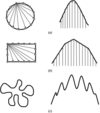Figure 10 The graphs of ordered distances from some starting point to every other point on the boundary in order. In (a) the boundary is a circle, in (b) a rectangle and in (c) a more complicated boundary, typical of those obtained for precipitate.

### 4. Object classification

Table 1summarizes the classifiers used in the present method. Each separate object in an image is evaluated in terms of these variables to obtain a vector of values associated with that object. These vectors are then used to assign each object to a particular class.

A training data set consisting of ∼200 objects from each class was used to provide probability distributions for the classifiers. The objects were then classified by eye and evaluated in terms of the classifiers that have been described. Thus, for each classifier, say Xi, a probability distribution can be obtained from the values of this variable for all the objects in the class XTAL, say. In other words, we have the conditional probability distribution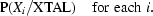Labelling the five classes Ck, for k = 1, …, 5, gives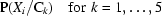for each i. Also, since the number of objects in each class is known, P(Ck) is known and so Bayes theorem (see Bayes, 1763) can be used to find the probability of an object being in any class given that it has a particular value of Xi. That is,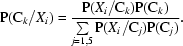Thus, each classifier gives the probability of an object being in a particular class. These probabilities are combined by assuming independence and simply multiplying them together to obtain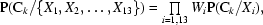where the Wi indicate weights. In the results of the next section, all the classifiers were given equal weights, although some may well prove to be more reliable than others and weighting schemes are now being tried. Weights would be accounted for automatically in a suitable neural network combination of the classifiers and such a system is also being considered.

### 5. Results

With the probability distributions obtained from a training data set, the algorithm was tested on new images. Initially, each object is assigned to a particular class based on the values obtained for the classifier variables. Fig. 11shows the results of this classification on test images. Here, the classes Xtal and PILE are combined as Xtal, as both classes indicate the presence of crystals. Also, objects from the class SKIN are shown in the class JUNK as all are unfavourable. The graph shows that 86% of crystals are identified correctly and that 77% of unfavourable objects are also classified correctly. However, objects belonging to the class INT, indicating promising conditions, are often classified incorrectly. In particular, some objects that could be of interest are classified as unfavourable. This reflects the difficult and subjective judgements made in the usual classification. Encouragingly, however, crystalline precipitate is usually identified correctly. Furthermore, the results presented are for individual objects and many of the wrongly classified objects are insignificant. The results are better when considered over an entire image.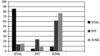Figure 11 The different greys represent the classes that the various objects tested actually belong to, as shown in the key. The graph shows how these objects were classified (as a percentage of the total number of objects in each class). For example, dark grey represents objects belonging to the class XTAL and the graph shows that 86% of these objects were classified correctly as crystals. It can be seen that the class INT of interesting objects causes most problems.

Currently, the number of objects assigned to each class is output as a percentage of pixels. These are percentages of the pixels in objects and not of the total number of pixels in the image. For example, if there is only one small crystal in the image, it will be classified as 100% crystals'. On the other hand, for a small crystal and lots of precipitate, a possible classification would be 10% crystals, 90% interesting'. A suitable scoring system still needs to be devised. The aim is to assign single scores to images indicating their significance.

Images from various sources have been tested. This does not present a problem, except that the initial parameters need to be adjusted to the expected resolution. In the images shown here, the crystallization drop approximately fills the image. Although this sometimes makes identification of the drop edge difficult, it is not important for these cases. However, for images in which the drop is very small in comparison to the overall image, it is much more important to identify the crystallization drop and eliminate everything outside it. In practice, the type and resolution of images for a particular setup would be sufficiently consistent.

The following three examples are cases in which the entire image is considered. Therefore, objects arising from the edge of the drop or the well will also be present and should be classified appropriately. Table 2shows the results as the number of objects from each class and the percentage of pixels in each class. Image 1 the table is shown in Fig. 12(a). In this case, a large percentage of the pixels have been identified as belonging to objects classified as piles of crystals. This is owing to crystals and precipitate being grouped together and considered as single objects (see Fig. 12b).

 The number of objects assigned to each class is given as well as the percentage of object pixels (i.e. only those pixels in objects, not all pixels in the image) assigned to each class. Image 1 is the image shown in Fig. 12and it can be seen that most objects have been classified as crystals or interesting objects. Image 2 is shown in Fig. 13and here a large pile of crystals has been identified. A large percentage of pixels have been classed as interesting owing to the area covered by the precipitate. Image 3 is shown in Fig. 14, with over 90% of the object pixels correctly classified as arising from skin.
Image Class No. of objects in each class % of pixels in each class
Crystal 4 15.56
Pile of crystals 2 39.59
Interesting 18 27.59
Skin 3 9.13
Junk 15 8.13

Crystal 0 0.00
Pile of crystals 1 35.85
Interesting 2 61.31
Skin 0 0.00
Junk 5 2.84

Crystal 0 0.00
Pile of crystals 0 0.00
Interesting 2 4.71
Skin 8 91.42
Junk 8 3.87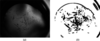Figure 12 The objects found for the image in (a) (image 1 in Table 2) are shown in (b). Notice that some crystals and precipitate have been amalgamated into single objects. These objects have been classified as `piles of crystals'.

The percentage of pixels in each class also indicates the size of the objects found. Image 2 from the table (Fig. 13) shows a large percentage of pixels classified as interesting. This is because the precipitate covers a large area in comparison to the pile of crystals. Fig. 14shows image 3 from Table 2, a less desirable effect owing to skin forming on the crystallization drop. Although a quantification scheme for the results still needs to be formulated, it can easily be seen from the graphs in Fig. 15the sort of outcomes the three images exhibit.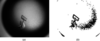Figure 13 The objects found for the image in (a) (image 2 in Table 2) are shown in (b). The precipitate covers a large area, explaining the percentages shown in Table 2.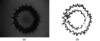Figure 14 The objects found for the image in (a) (image 3 in Table 2) are shown in (b). Although the objects exhibit straight boundary sections and regular angles, it is immediately obvious from the shapes that the objects are not crystals.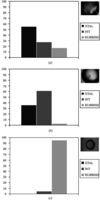Figure 15 (a), (b) and (c) show the graphs of results associated with Figs. 12, 13and 14, respectively. The results are also given in Table 2. It can be seen that the first two images have been classified as having crystals, whereas the third image is mostly rubbish.

Further classifiers and weighting schemes are being investigated as well as different classification methods such as neural networks and cluster analysis. No automated image-recognition system can be expected to identify objects with complete accuracy, but one that errs on the side of caution and produces few false negatives would dramatically reduce the amount of human intervention necessary. With robots capable of performing tens of thousands of crystallization experiments a day, effective automated image classification is essential.

### Acknowledgements

The author would like to thank Victor Lamzin, EMBL Hamburg for useful discussion and for supplying the first images used in this research. The author is a Royal Society University Research Fellow and would like to acknowledge the support of the Royal Society.

### ReferencesBIOLOGICALCRYSTALLOGRAPHY